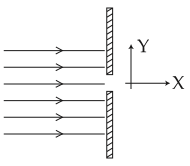#### A parallel beam of electrons travelling in x-direction falls on a slit of width d (see figure). If after passing the slit, an electron acquires momentum py in the y-direction then for a majority of electrons passing through the slit (h is Plancks constant) :Option 1)Option 2)Option 3)Option 4)As we learnt in

Fraunhofer Diffraction -- wherein

Condition of nth minima.slit widthangle of deviationCorrect option is 3.

Option 1)This is an incorrect option.

Option 2)This is an incorrect option.

Option 3)This is the correct optopn.

Option 4)This is an incorrect option.

#### Plabita